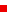## ISR Events CalendarEvent Information Numerical Analysis Seminar: Nicholas Higham, "Exploiting Multiprecision Arithmetic" Tuesday, October 30, 2018 3:30 p.m. 3206 Kirwan Hall For More Information: Howard Elman ################################################################### N U M E R I C A L   A N A L Y S I S   S E M I N A R Department of Mathematics, UMIACS and IPST ################################################################### Exploiting Multiprecision Arithmetic *Nicholas J. Higham* *School of Mathematics, Manchester University* Abstract: There is a growing availability of multiprecision arithmetic: floating point arithmetic in multiple, possibly arbitrary, precisions. Demand in applications includes for both low precision (deep learning and climate modelling) and high precision (long-term simulations and solving very ill conditioned problems). We discuss - Half-precision arithmetic: its characteristics, availability, attractions, pitfalls, and rounding error analysis implications. - Quadruple precision arithmetic: the need for it in applications, its cost, and how to exploit it. As an example of the use of multiple precisions we discuss iterative refinement for solving linear systems. We explain the benefits of combining three different precisions of arithmetic (say, half, single, and double) and show how a new form of preconditioned iterative refinement can be used to solve very ill conditioned sparse linear systems to high accuracy. ################################################################### The Numerical Analysis Seminar is sponsored by the Department of Mathematics, the Institute for Physical Science and Technology and the University of Maryland Institute for Advanced Computer Studies; University of Maryland, College Park. For further information, contact the seminar organizers: Howard Elman elman@cs.umd.edu Ricardo H. Nochetto rhn@math.umd.edu Juan Pablo Borthagaray jpb@umd.edu For more information about upcoming talks, see  http://www-math.umd.edu/research/seminars/numerical-analysis-seminar.html ###################################################################Browse Events By Calendar
July 2019
SU M TU W TH F SA
1 2 3 4 5 6 w
7 8 9 10 11 12 13 w
14 15 16 17 18 19 20 w
21 22 23 24 25 26 27 w
28 29 30 31 w

Search Events

ISR lecture and seminar series